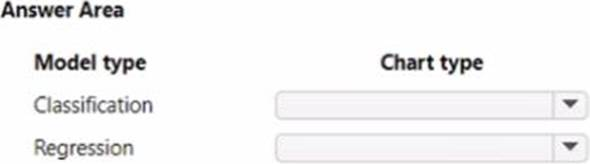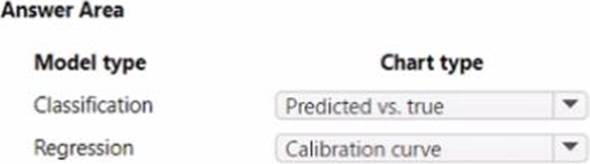## You manage an Azure Machine Learning workspace. You create an experiment named experiment1 by using the Azure Machine Learning Python SDK v2 and MLflow

HOTSPOT

You manage an Azure Machine Learning workspace. You create an experiment named experiment1 by using the Azure Machine Learning Python SDK v2 and MLflow.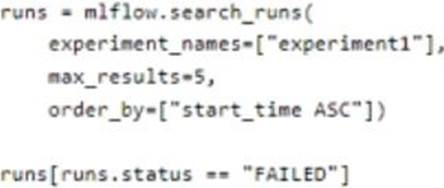For each of the following statements, select Yes if the statement rs true. Otherwise, select No.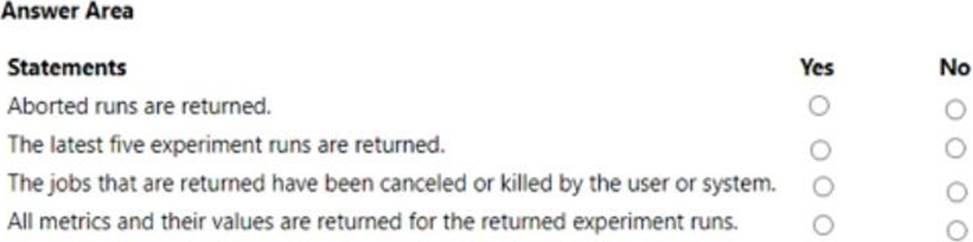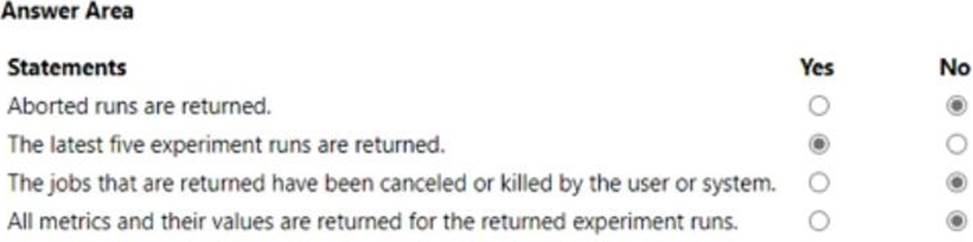## How should you complete the AutoMLConfig definition?

HOTSPOT

You collect data from a nearby weather station.

You have a pandas dataframe named weather_df that includes the following data:The data is collected every 12 hours: noon and midnight.

You plan to use automated machine learning to create a time-series model that predicts temperature over the next seven days. For the initial round of training, you want to train a

maximum of 50 different models.

You must use the Azure Machine Learning SDK to run an automated machine learning experiment to train these models.

You need to configure the automated machine learning run.

How should you complete the AutoMLConfig definition? To answer, select the appropriate options in the answer area. NOTE: Each correct selection is worth one point.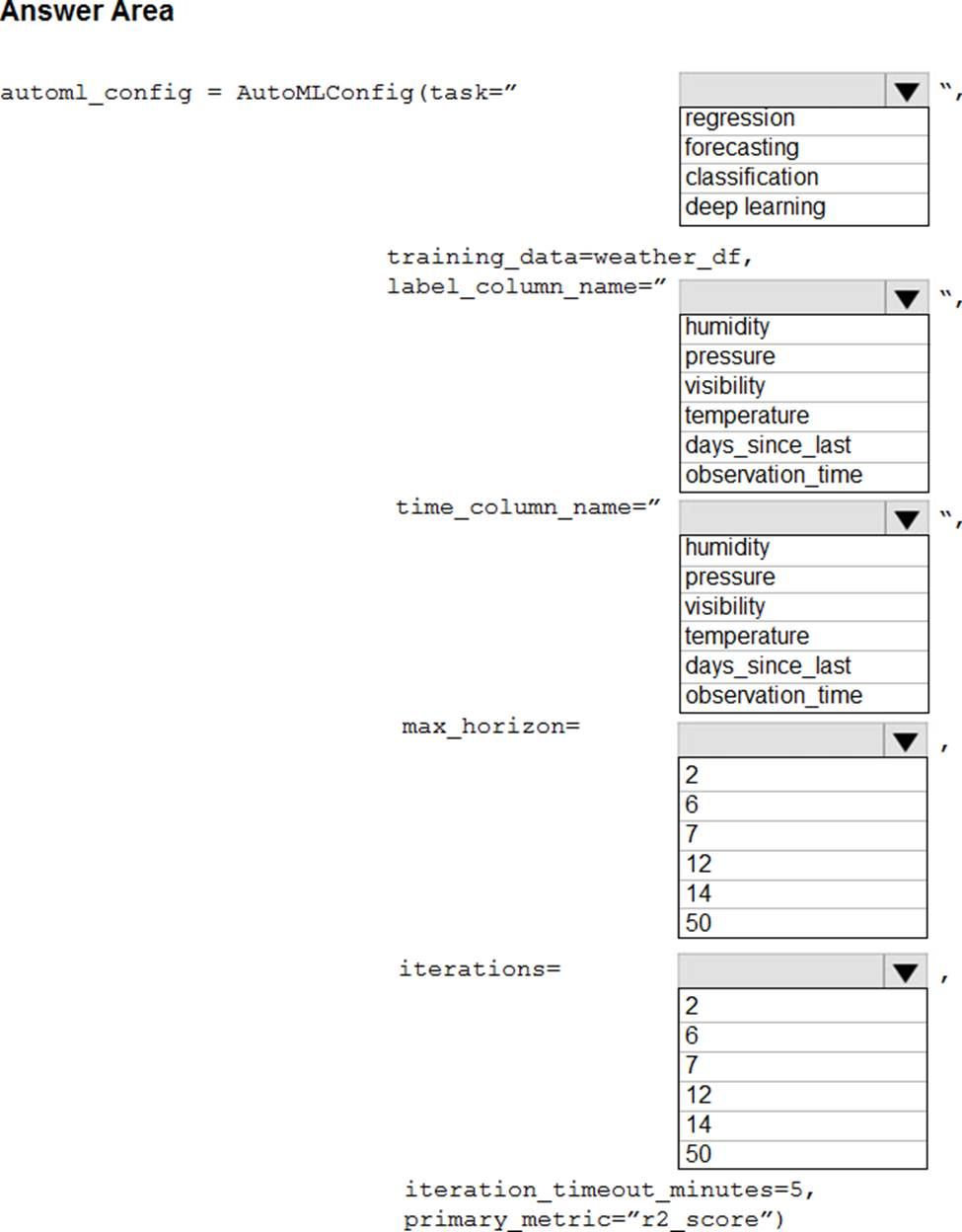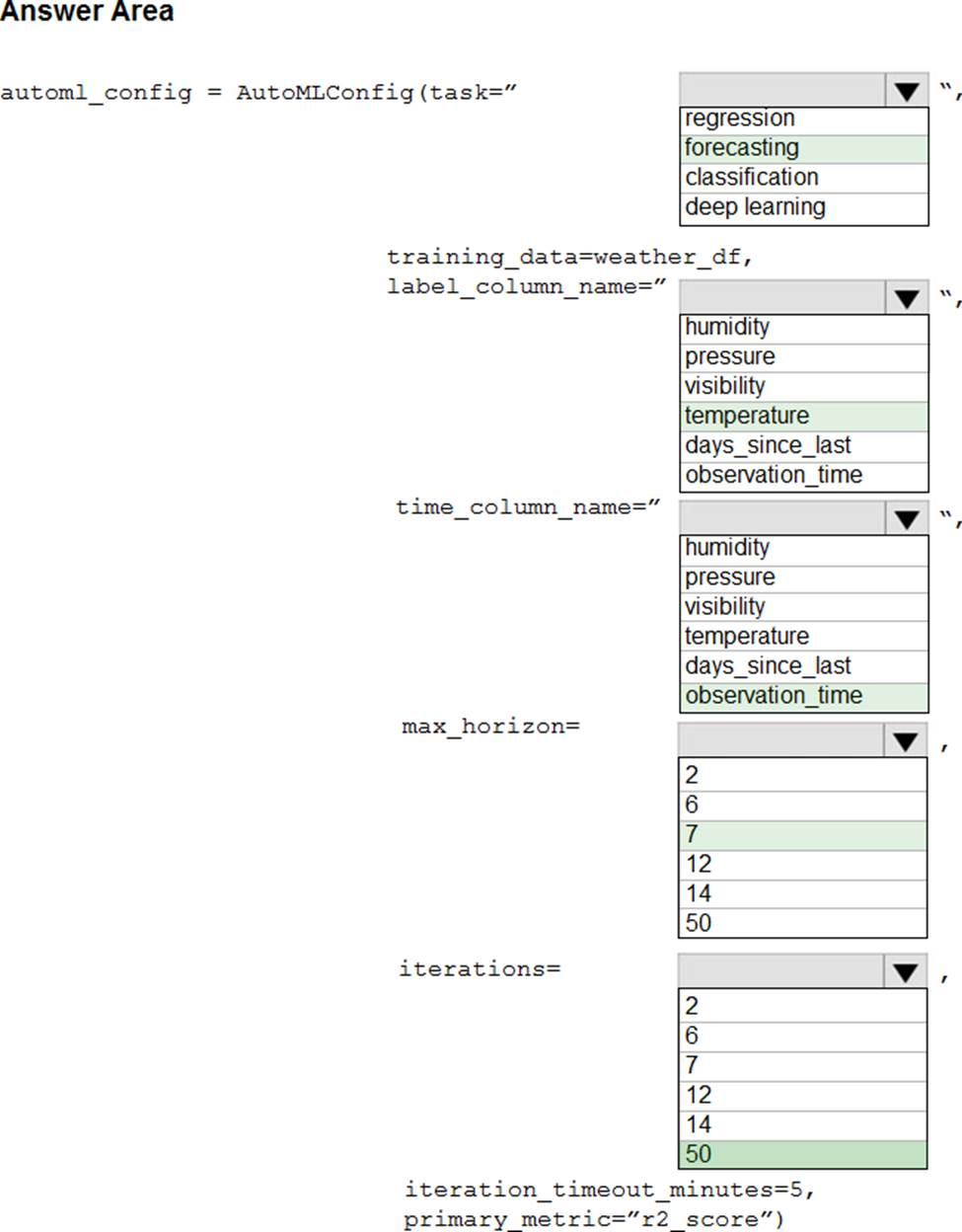Explanation:

Box 1: forcasting

Task: The type of task to run. Values can be ‘classification’, ‘regression’, or ‘forecasting’ depending on the type of automated ML problem to solve.

Box 2: temperature

The training data to be used within the experiment. It should contain both training features and a label column (optionally a sample weights column).

Box 3: observation_time

time_column_name: The name of the time column. This parameter is required when forecasting to specify the datetime column in the input data used for building the time series and inferring its frequency. This setting is being deprecated. Please use forecasting_parameters instead.

Box 4: 7

"predicts temperature over the next seven days"

max_horizon: The desired maximum forecast horizon in units of time-series frequency. The default value is 1.

Units are based on the time interval of your training data, e.g., monthly, weekly that the forecaster should predict out. When task type is forecasting, this parameter is required.

Box 5: 50

"For the initial round of training, you want to train a maximum of 50 different models."

Iterations: The total number of different algorithm and parameter combinations to test during an automated ML experiment.

## Which development technique should you use?

HOTSPOT

You plan to implement an Azure Machine Learning solution.

You have the following requirements:

• Run a Jupyter notebook to interactively tram a machine learning model.

• Deploy assets and workflows for machine learning proof of concept by using scripting rather than custom programming.

You need to select a development technique for each requirement

Which development technique should you use? To answer, select the appropriate options in the answer area. NOTE: Each correct selection is worth one point.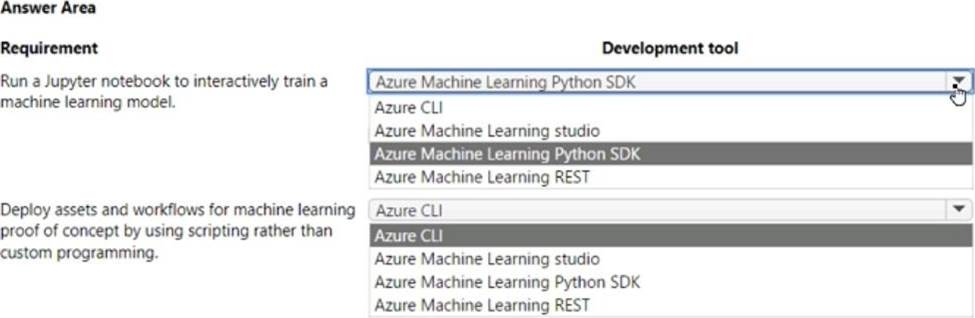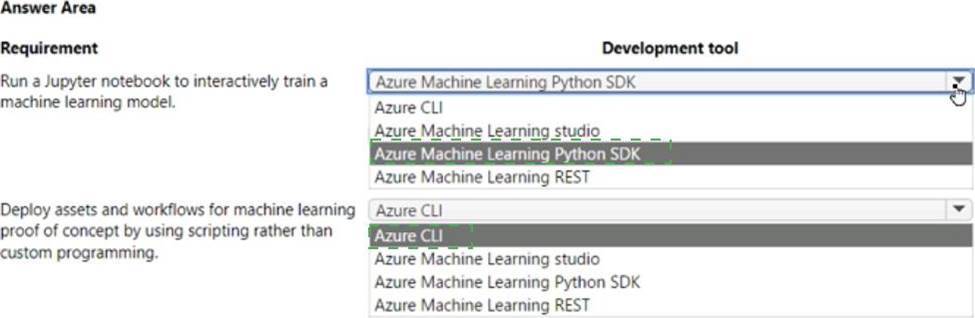## Does the solution meet the goal?

Note: This question is part of a series of questions that present the same scenario. Each question in the series contains a unique solution that might meet the stated goals. Some question sets might have more than one correct solution, while others might not have a correct solution.

After you answer a question in this section, you will NOT be able to return to it. As a result, these questions will not appear in the review screen.

You are creating a new experiment in Azure Machine Learning Studio.

One class has a much smaller number of observations than the other classes in the training set.

You need to select an appropriate data sampling strategy to compensate for the class imbalance.

Solution: You use the Scale and Reduce sampling mode.

Does the solution meet the goal?
A . Yes
B. No

Explanation:

Instead use the Synthetic Minority Oversampling Technique (SMOTE) sampling mode.

Note: SMOTE is used to increase the number of underepresented cases in a dataset used for machine learning. SMOTE is a better way of increasing the number of rare cases than simply duplicating existing cases.

References: https://docs.microsoft.com/en-us/azure/machine-learning/studio-module-reference/smote

## Which properties should you select?

HOTSPOT

You need to identify the methods for dividing the data according, to the testing requirements.

Which properties should you select? To answer, select the appropriate option-, m the answer area. NOTE: Each correct selection is worth one point.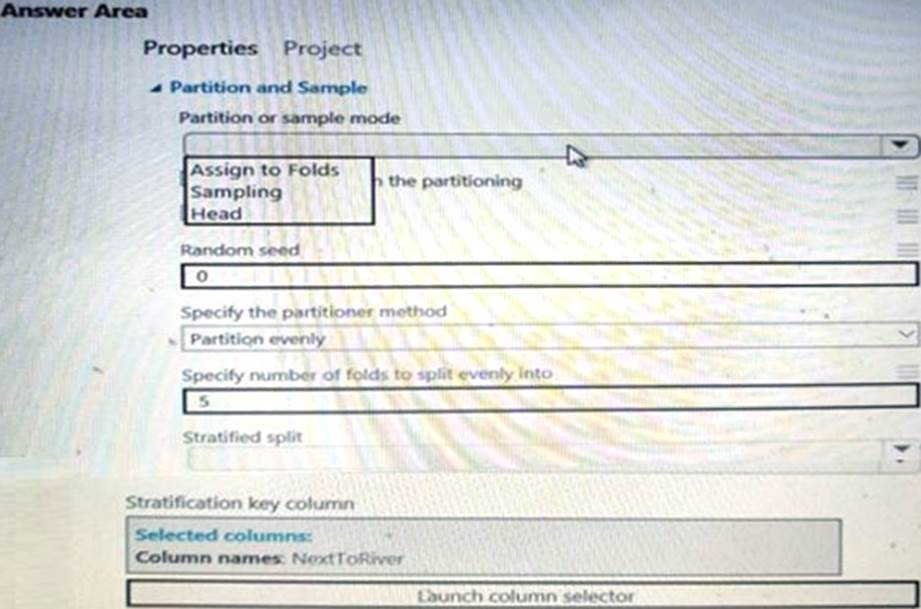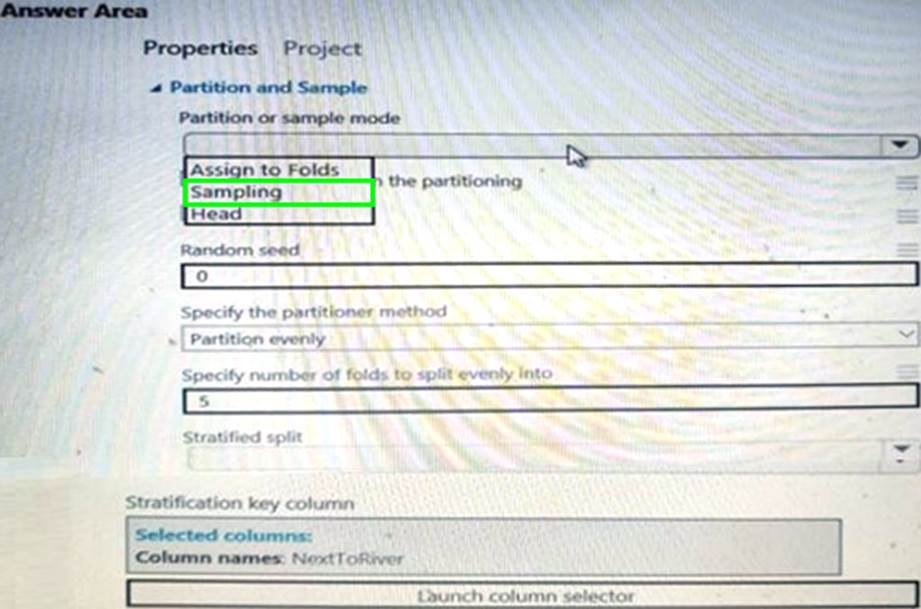## How should you complete the code segment?

HOTSPOT

You need to use the Python language to build a sampling strategy for the global penalty detection models.

How should you complete the code segment? To answer, select the appropriate options in the answer area. NOTE: Each correct selection is worth one point.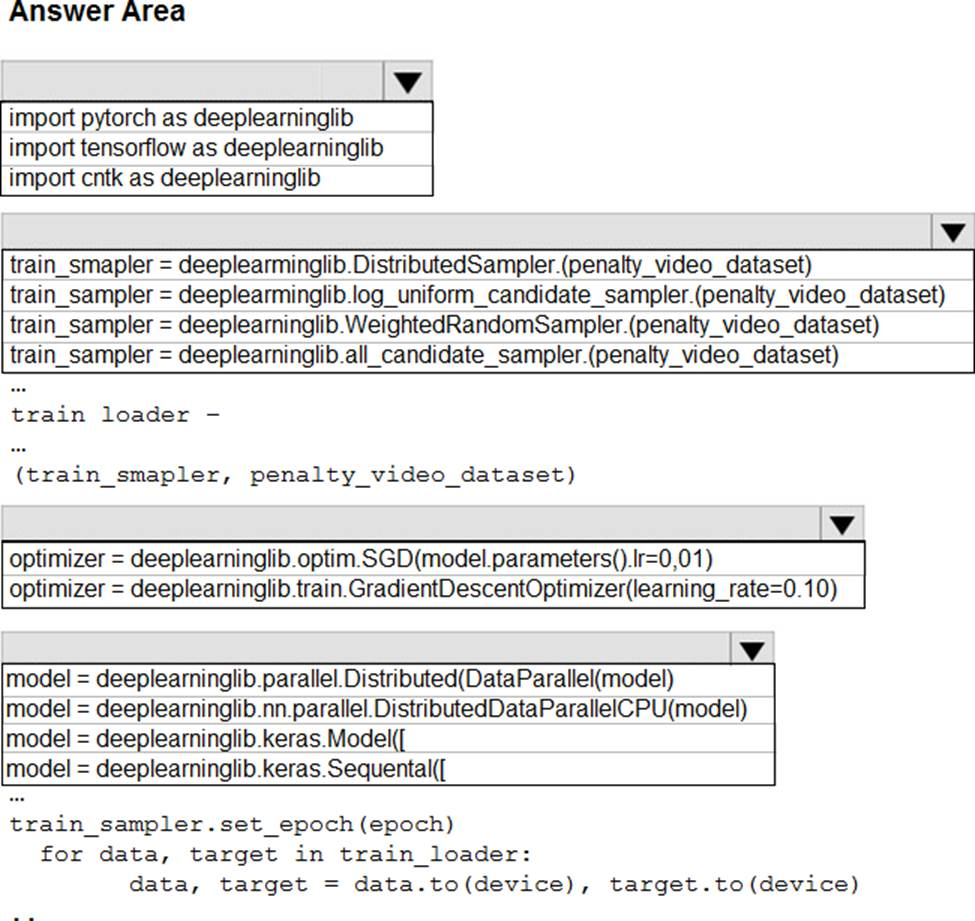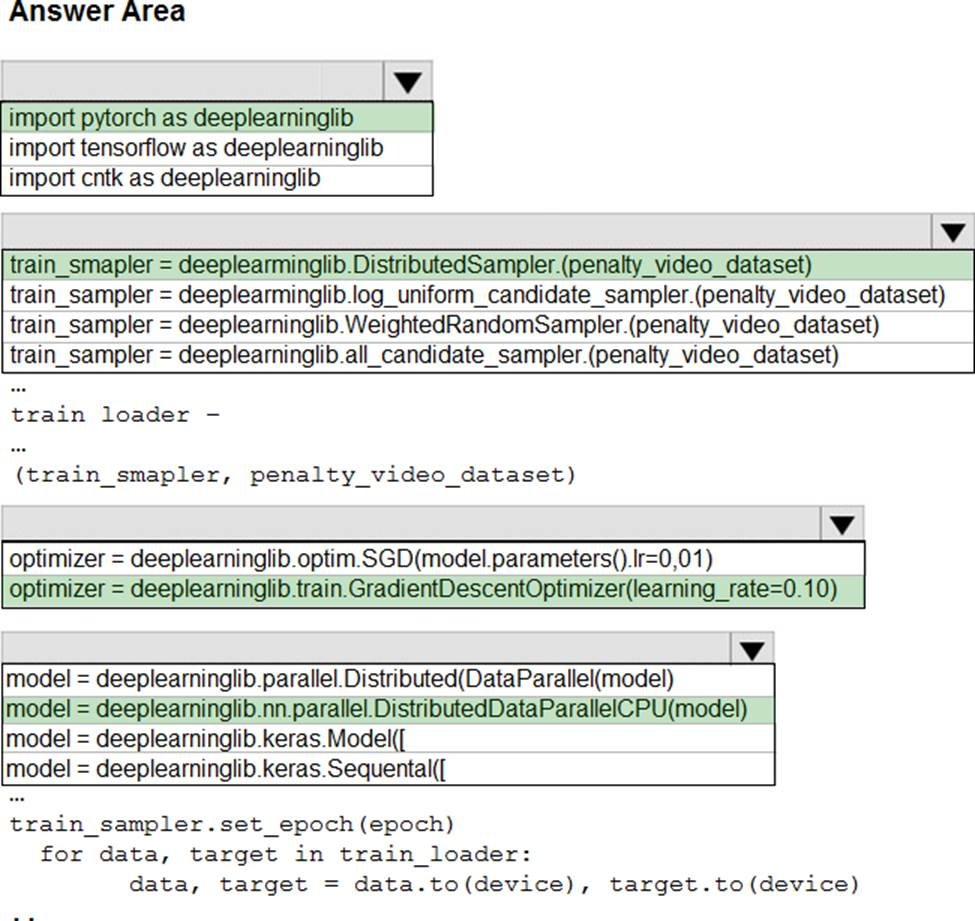Explanation:

Box 1: import pytorch as deeplearninglib

Box 2: ..DistributedSampler(Sampler)..

DistributedSampler(Sampler):

It is especially useful in conjunction with class:`torch.nn.parallel.DistributedDataParallel`. In such case, each process can pass a DistributedSampler instance as a DataLoader sampler, and load a subset of the original dataset that is exclusive to it.

Scenario: Sampling must guarantee mutual and collective exclusively between local and global segmentation models that share the same features.

Box 3: optimizer = deeplearninglib.train. GradientDescentOptimizer(learning_rate=0.10)

## Which code should you use to configure the estimator?

You create a datastore named training_data that references a blob container in an Azure Storage account. The blob container contains a folder named csv_files in which multiple comma-separated values (CSV) files are stored.

You have a script named train.py in a local folder named ./script that you plan to run as an experiment using an estimator.

The script includes the following code to read data from the csv_files folder: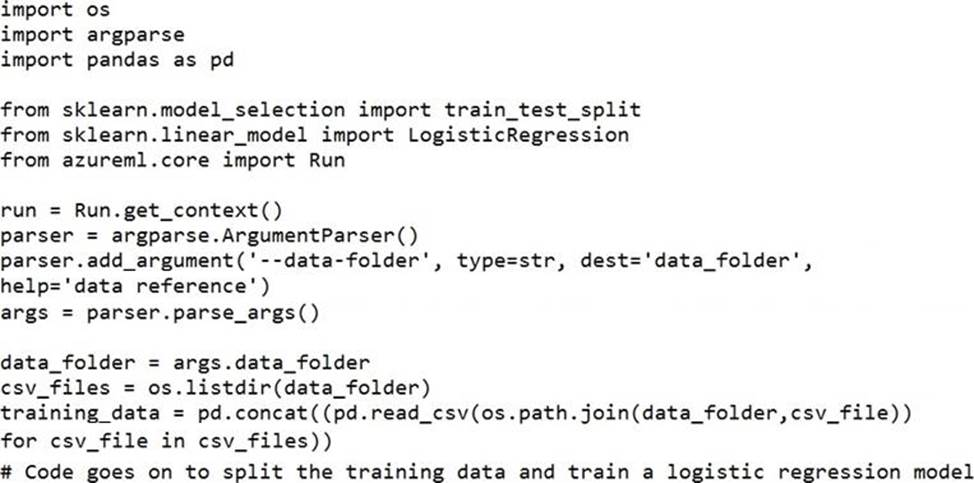You have the following script.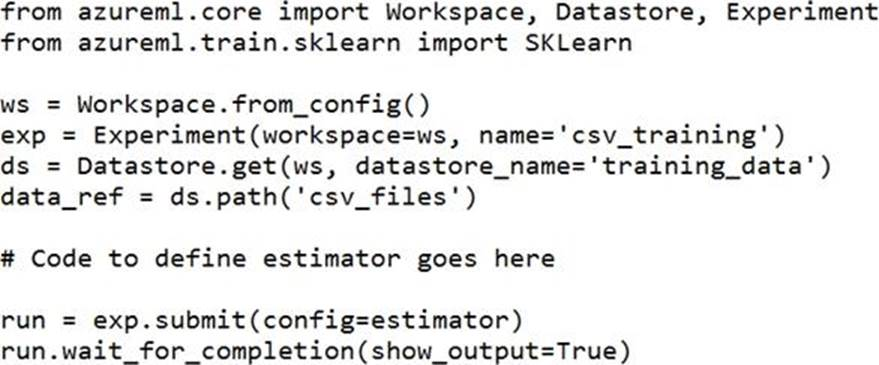You need to configure the estimator for the experiment so that the script can read the data from a data reference named data_ref that references the csv_files folder in the training_data datastore.

Which code should you use to configure the estimator?

A)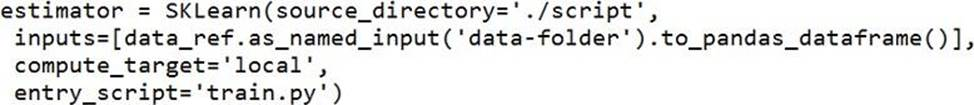B)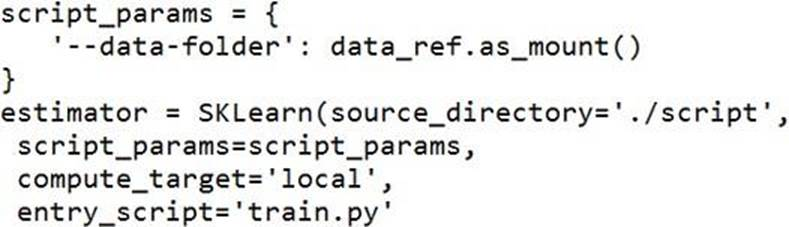C)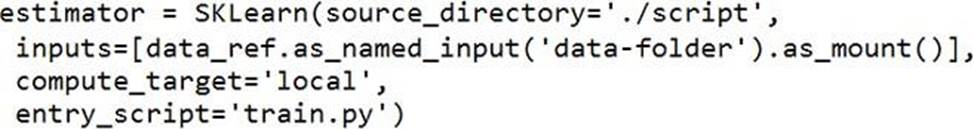D)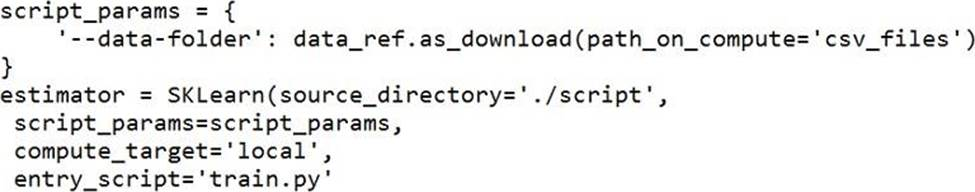E)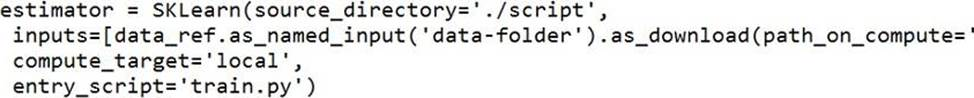A . Option A
B. Option B
C. Option C
D. Option D
E. Option E

Explanation:

Besides passing the dataset through the inputs parameter in the estimator, you can also pass the dataset through script_params and get the data path (mounting point) in your training script via arguments. This way, you can keep your training script independent of azureml-sdk. In other words, you will be able use the same training script for local debugging and remote training on any cloud platform.

Example:

from azureml.train.sklearn import SKLearn

script_params = {

# mount the dataset on the remote compute and pass the mounted path as an argument to the training script

‘–data-folder’: mnist_ds.as_named_input(‘mnist’).as_mount(),

‘–regularization’: 0.5

}

est = SKLearn(source_directory=script_folder,

script_params=script_params,

compute_target=compute_target,

environment_definition=env,

entry_script=’train_mnist.py’)

# Run the experiment

run = experiment.submit(est)

run.wait_for_completion(show_output=True)

A: Pandas DataFrame not used.

Reference: https://docs.microsoft.com/es-es/azure/machine-learning/how-to-train-with-datasets

## Which configurations should you use?

HOTSPOT

You create an Azure Machine Learning dataset. You use the Azure Machine Learning designer to transform the dataset by using an Execute Python Script component and custom code.

You must upload the script and associated libraries as a script bundle.

You need to configure the Execute Python Script component.

Which configurations should you use? To answer, select the appropriate options in the answer area. NOTE Each correct selection is worth one point.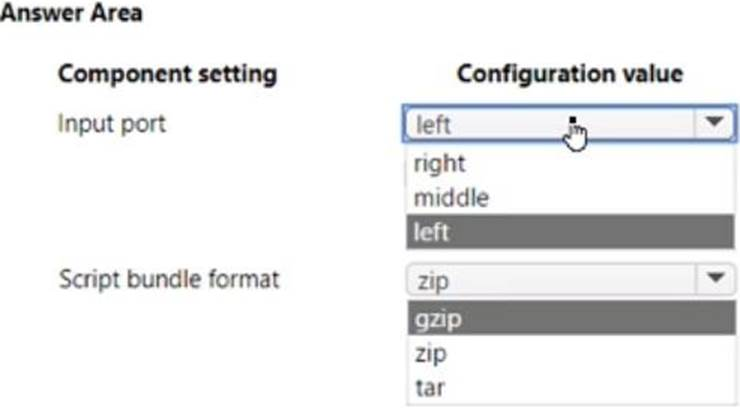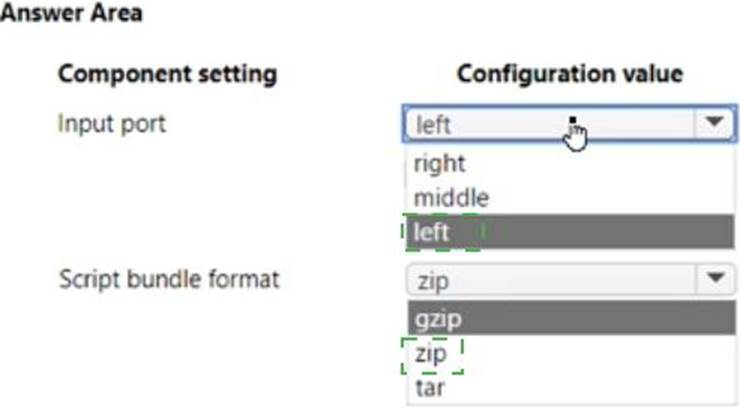## Which explainer modules should you use?

HOTSPOT

You are hired as a data scientist at a winery. The previous data scientist used Azure Machine Learning.

You need to review the models and explain how each model makes decisions.

Which explainer modules should you use? To answer, select the appropriate options in the answer area. NOTE: Each correct selection is worth one point.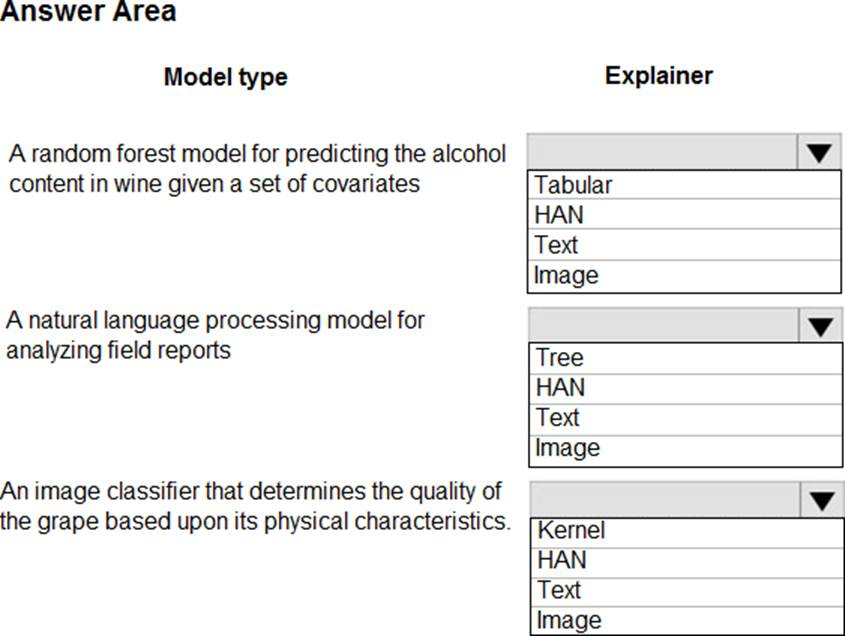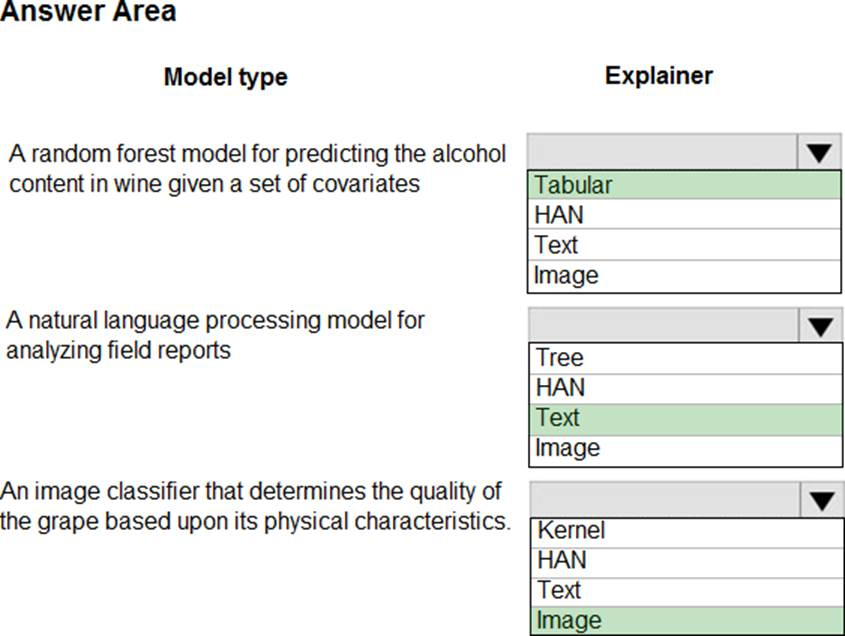Explanation:

Meta explainers automatically select a suitable direct explainer and generate the best explanation info based on the given model and data sets. The meta explainers leverage all the libraries (SHAP, LIME, Mimic, etc.) that we have integrated or developed.

The following are the meta explainers available in the SDK:

Tabular Explainer: Used with tabular datasets.

Text Explainer: Used with text datasets.

Image Explainer: Used with image datasets.

Box 1: Tabular

Box 2: Text

Box 3: Image

## Which chart types should you use?

CORRECT TEXT

You train classification and regression models by using automated machine learning.

You must evaluate automated machine learning experiment results. The results include how a classification model is making systematic errors in its predictions and the relationship between the target feature and the regression model’s predictions. You must use charts generated by automated machine learning.

You need to choose a chart type for each model type.

Which chart types should you use? To answer, select the appropriate options in the answer area. NOTE: Each correct selection is worth one point.